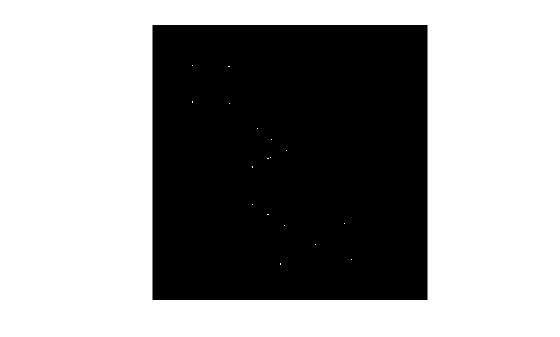bwulterode

Ultimate erosion

Description

example

BW2 = bwulterode(BW) computes the ultimate erosion of the binary image BW. The ultimate erosion of BW consists of the regional maxima of the Euclidean distance transform of the complement of BW.

BW2 = bwulterode(BW,method) specifies the distance transform method.

BW2 = bwulterode(___,conn) specifies the pixel connectivity.

Examples

collapse all

Read a binary image into the workspace and display it.

imshow(originalBW)Perform the ultimate erosion of the image and display it.

ultimateErosion = bwulterode(originalBW);
figure, imshow(ultimateErosion)Input Arguments

collapse all

Binary image, specified as a numeric or logical array of any dimension. For numeric input, any nonzero pixels are considered to be 1 (true).

Data Types: single | double | int8 | int16 | int32 | int64 | uint8 | uint16 | uint32 | uint64 | logical

Distance transform method, specified as one of the values in this table.

Method

Description

'chessboard'

In 2-D, the chessboard distance between (x1,y1) and (x2,y2) is

max(│x1x2│,│y1y2│).

'cityblock'

In 2-D, the cityblock distance between (x1,y1) and (x2,y2) is

x1x2│ + │y1y2

'euclidean'

In 2-D, the Euclidean distance between (x1,y1) and (x2,y2) is

$\sqrt{{\left({x}_{1}-{x}_{2}\right)}^{2}+{\left({y}_{1}-{y}_{2}\right)}^{2}}.$

'quasi-euclidean'

In 2-D, the quasi-Euclidean distance between (x1,y1) and (x2,y2) is

Pixel connectivity, specified as one of the values in this table. The default connectivity is 8 for 2-D images, and 26 for 3-D images.

Value

Meaning

Two-Dimensional Connectivities

4-connected

Pixels are connected if their edges touch. The neighborhood of a pixel are the adjacent pixels in the horizontal or vertical direction.8-connected

Pixels are connected if their edges or corners touch. The neighborhood of a pixel are the adjacent pixels in the horizontal, vertical, or diagonal direction.Three-Dimensional Connectivities

6-connected

Pixels are connected if their faces touch. The neighborhood of a pixel are the adjacent pixels in:

• One of these directions: in, out, left, right, up, and down18-connected

Pixels are connected if their faces or edges touch. The neighborhood of a pixel are the adjacent pixels in:

• One of these directions: in, out, left, right, up, and down

• A combination of two directions, such as right-down or in-up26-connected

Pixels are connected if their faces, edges, or corners touch. The neighborhood of a pixel are the adjacent pixels in:

• One of these directions: in, out, left, right, up, and down

• A combination of two directions, such as right-down or in-up

• A combination of three directions, such as in-right-up or in-left-downFor higher dimensions, bwulterode uses the default value conndef(ndims(BW),'maximal').

Connectivity can also be defined in a more general way for any dimension by specifying a 3-by-3-by- ... -by-3 matrix of 0s and 1s. The 1-valued elements define neighborhood locations relative to the center element of conn. Note that conn must be symmetric about its center element. See Specifying Custom Connectivities for more information.

Data Types: double | logical

Output Arguments

collapse all

Eroded image, returned as a logical array of the same size as BW.

Data Types: logical